Latest Banking jobs   »

# Quantitative Aptitude Quiz For Bank of Baroda AO 2023 -8th March

Directions (1-5): In each of these questions, two equations (I) and (II) are given. Solve the equations and mark the correct option: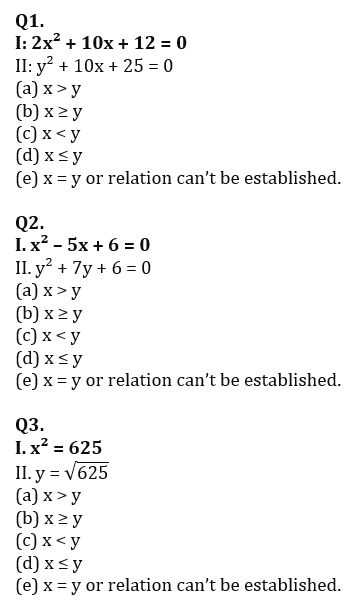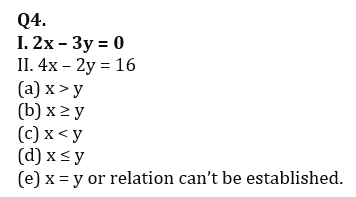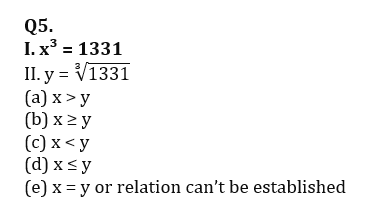Directions (6-10):- In each of the following questions, two equations (I) and (II) are given. Solve the equations and mark the correct option:
(a) if x>y
(b) if x≥y
(c) if x<y
(d) if x ≤y
(e) if x = y or no relation can be established between x and y.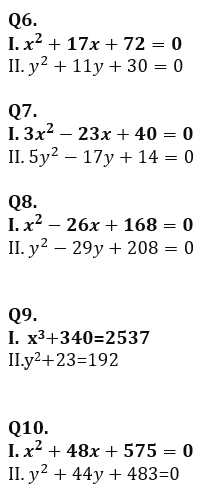Directions (11-15):- In each of the following questions, two equations (I) and (II) are given. Solve the equations and mark the correct option:
(a) if x>y
(b) if x≥y
(c) if x<y
(d) if x ≤y
(e) if x = y or no relation can be established between x and y.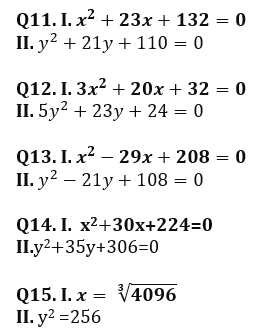Solutions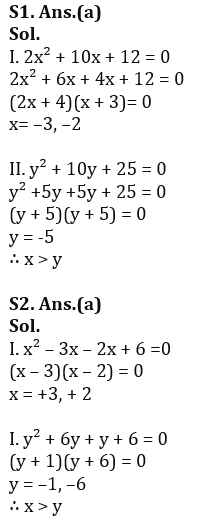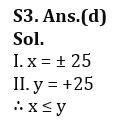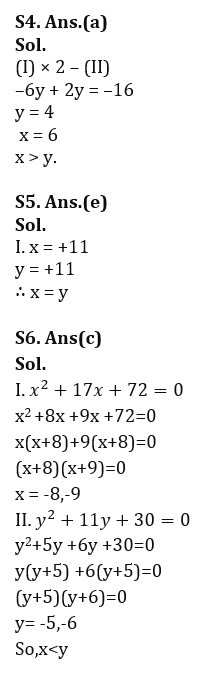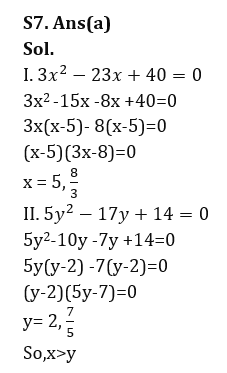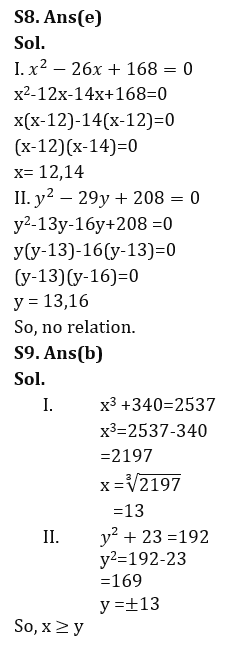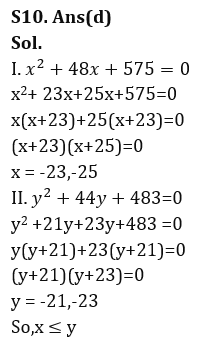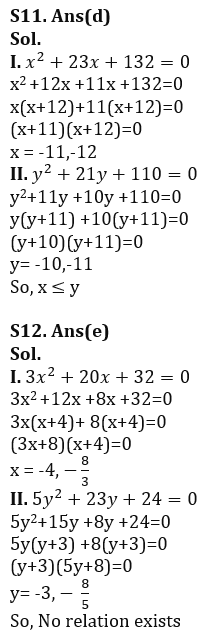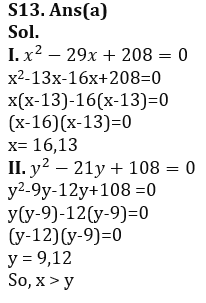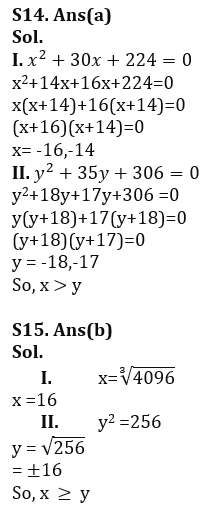.                                                               .                                                                                                                                                                                                     .

## FAQs

### What is the selection process of Bank of Baroda AO 2023?

The selection process of Bank of Baroda AO consists of Online exam and Interview

#### Congratulations!Union Budget 2023-24: Free PDF